This example shows how to estimate the global positioning system (GPS) receiver position using a multi-satellite GPS baseband waveform. You use the receiver independent exchange format (RINEX) and an almanac file to model the GPS constellation and generate a multi-satellite baseband waveform. Simulate the satellite scenario to get relative positions of satellites with respect to the modeled receiver. For this satellite scenario, model the Doppler shift, delay, and received signal power. Based on these calculations, impair the generated baseband signal with Doppler shift, delay, and noise. This example shows how to estimate the simulated receiver position from this impaired GPS baseband signal.

### Introduction

In this example, you start with a RINEX file and use `rinexread` (Navigation Toolbox) to read the file and provide input to `satelliteScenario` (Satellite Communications Toolbox) to simulate the GPS constellation. Based on this GPS constellation and a given receiver position, you calculate the Doppler shift, delay, and signal path loss from each visible satellite to the receiver. Based on the ephemeris and clock and almanac data from the RINEX and almanac files, generate data bits as per the IS-GPS-200  standard. For the satellites that are visible to the receiver, generate coarse acquisition code (C/A-code) and precision code (P-code). P-code is placed on the in-phase (I) branch and C/A-code is placed on the quadrature-phase (Q) branch of the baseband waveform. The I-branch signal is attenuated by 3 dB as given in IS-GPS-200 . Generate the baseband waveform for all the visible satellites and pass this baseband waveform through the propagation channel as shown in the following figure. Propagation channel characteristics for each satellite signal are unique because the position and velocity of each satellite with respect to the receiver is unique. In this example, you model the characteristics of the propagation channel — namely, the Doppler shift, delay, and scaling signal power based on the propagation path loss — and add thermal noise to the composite signal. Provide this noisy signal as input to the GPS receiver. This example supports storing this noisy signal in a file so that you can test your receiver.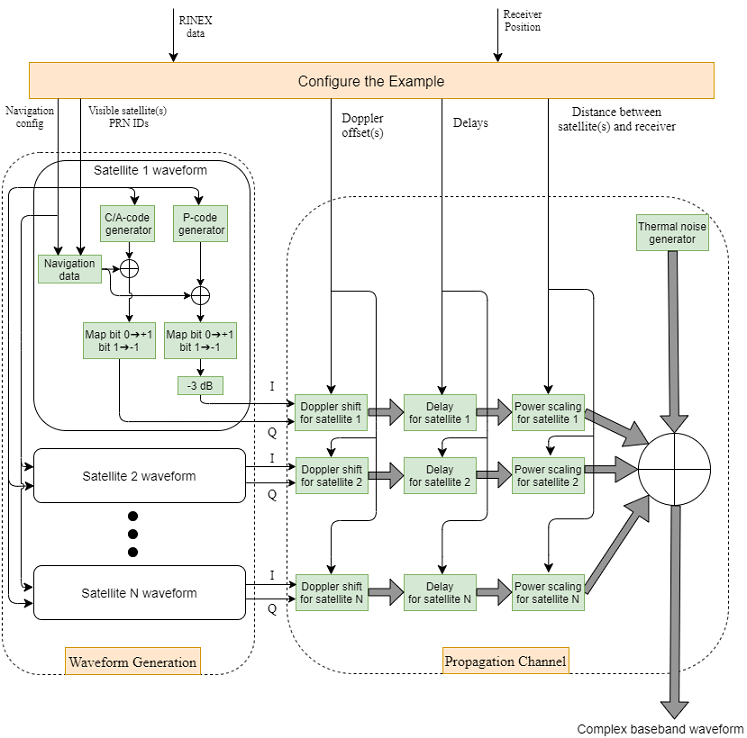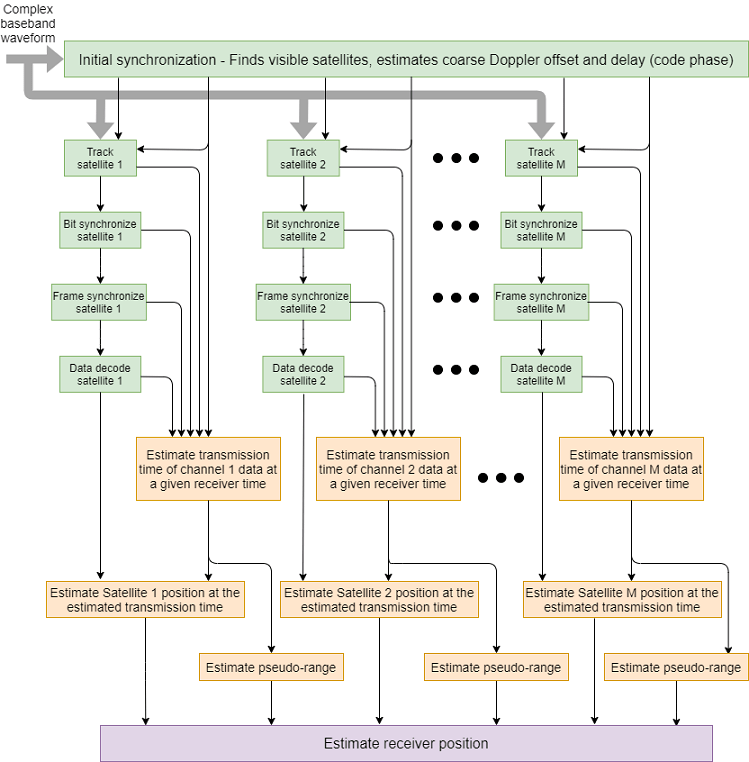### Initialize Parameters

Initialize the parameters that are necessary to configure and run the end-to-end GPS receiver simulation.

Initialize the data duration for which this example must run. Typically, a GPS receiver needs at least 50 seconds of data to estimate the receiver position. To simulate this example for 50 seconds of data would take a lot of wall-clock time based on the available computer resources. For the purposes of this example, set the data duration to 3 seconds. Also, initialize the sampling rate for baseband waveform generation.

```simulatedDataDuration = 3; % In seconds samplingRate = 10.23e6; % In Hz```

If needed, enable the `WriteWaveformToFile` property so that you can process this waveform through the receiver of your choice.

`WriteWaveformToFile =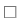false;`

This example processes data in chunks of 1 millisecond. That is, 1 millisecond of data is generated by the waveform generator and that 1 millisecond of data is processed by the receiver. This example works for a step time of one millisecond only.

```stepTime = 1e-3; % In seconds numSteps = (simulatedDataDuration/stepTime) + 1;```

Initialize the parameters required to set up the channel. This example generates a GPS waveform from a simulated GPS constellation. As a starting point to simulate the GPS constellation, read the RINEX file.

```rinexFileName = "GODS00USA_R_20211750000_01D_GN.rnx"; almanacFileName = "gpsAlmanac.txt"; rinexdata = rinexread(rinexFileName);```

Initialize the receiver position to model the propagation channel based on its location. To estimate the receiver position, model the propagation delay accurately. The delay depends on the receiver position. This example models a stationary receiver and does not support a moving receiver.

```rxlat = 39.021; % Latitude in degrees north rxlon = -76.827; % Longitude in degrees east; negative longitude is degrees west rxel = 19; % Elevation in meters```

Because the GPS data bit rate is 50 bits per second and each C/A-code block is 1 millisecond, each bit consists of 20 C/A-code blocks. Initialize this parameter.

`numCACodeBlocksPerBit = 20;`

Initialize the properties required for the receiver. The Receiver must wait for some time before it can start receiving some meaningful data because of large delays modeled in the example. A typical GPS signal has a delay of 60 to 90 milliseconds. So, if receiver waits for 100 milliseconds, then it starts to process signals rather than pure noise.

```rxWaitTime = 100; % Milliseconds performInitSync = true; % Initially this must be set to true % Initialize maximum number of tracking channels. Minimum of 4 tracking % channels are needed for proper functioning of the GPS receiver. maxNumTrackingChannels = 8; % Noise bandwidth of each of the tracking loops PLLNoiseBW = 90; % In Hz FLLNoiseBW = 4; % In Hz DLLNoiseBW = 3; % In Hz % Bit synchronization parameters isBitSyncComplete = zeros(maxNumTrackingChannels,1); numBitsForBitSync = 100; numWaitingStepsForBitSync = numCACodeBlocksPerBit*numBitsForBitSync; rxcntr = 1;```

Position estimation requires, at minimum, that subframes 2 and 3 are decoded. The length of a subframe is 6 seconds. For this example, decode 48.5 seconds of data to ensure that subframes 2 and 3 are included.

```minTimeForPosEst = 48.5; % In seconds minStepsForPosEst = minTimeForPosEst/stepTime; subframeDuration = 6; % In seconds numStepsPerSubframe = subframeDuration/stepTime;```

Initialize the physical constants required for the simulation.

```c = physconst("LightSpeed"); % Speed of light in m/sec fe = 1575.42e6; % GPS L1 frequency in Hz Dt = 12; % Directivity of the transmit antenna in dBi DtLin = db2pow(Dt); Dr = 4; % Directivity of the receive antenna in dBi DrLin = db2pow(Dr); Pt = 44.8; % Typical transmission power of a GPS satellite in watts k = physconst("boltzmann"); % Boltzmann constant in Joules/Kelvin T = 300; % Room temperature in Kelvin rxBW = 24e6; % Bandwidth in Hz Nr = k*T*rxBW; % Thermal noise power in watts rng default; % Initializing to default random number generation```

### Simulation Configuration

In this section, configure the example based on the parameters that are initialized in the Initialize Parameters section.

Set up the satellite scenario based on the RINEX file.

```% Initialize satellite scenario sc = satelliteScenario; % Set up the satellites based on the RINEX data sat = satellite(sc,rinexdata,"OrbitPropagator","gps"); rx = groundStation(sc,rxlat,rxlon); % Set up the receiver ac = access(sat,rx); % Calculate access between satellites and the receiver % Get the list of satellites that are considered in satellite scenario from % the RINEX data indices = ones(length(sat),1); for isat = 1:length(sat) ele = orbitalElements(sat(isat)); % Check for match of time of applicability and pseudo-random noise % (PRN) IDs so that data from RINEX file is considered for waveform % generation indices(isat) = find(rinexdata.GPS(:,:).Toe == ele.GPSTimeOfApplicability & ... rinexdata.GPS(:,:).SatelliteID == ele.PRN); end```

Generate the navigation data to transmit. First generate a navigation configuration object using the helper function `HelperGPSRINEX2Config`. This helper function maps the parameters read from the RINEX file to the configuration parameters needed for navigation data generation.

```% Generate navigation configuration object navcfg = HelperGPSRINEX2Config(almanacFileName,rinexdata.GPS(indices,:)); [mintow,locmintow] = min([navcfg(:).HOWTOW]); % The time of week (TOW) value is such that it must be 1 plus a multiple of % 5 so that data of subframe 1 is always generated first. mintow = ceil((mintow-1)/5)*5 + 1; % HOWTOW is a counter that contains integer values. Incrementing by a value % of 1 represents 6 seconds of data. The counter increases by a value of 1 % for each new subframe. [navcfg(:).HOWTOW] = deal(mintow); % Set the starting of frames based on mintow firstsubframeID = mod(mintow-1,125) + 1; frameID = ceil(firstsubframeID/5); allFrameIDs = [frameID:25,1:(frameID-1)]; [navcfg(:).FrameIndices] = deal(allFrameIDs); % Generate GPS navigation data numNavBits = 37500; % Full GPS data length is 37500 bits navdata = zeros(numNavBits,length(navcfg)); for isat = 1:length(navcfg) navdata(:,isat) = HelperGPSNAVDataEncode(navcfg(isat)); end```

Get the satellite positions and velocities over time for accurate modeling of the Doppler, delay, and power. For more information, see Calculate Latency and Doppler in a Satellite Scenario (Satellite Communications Toolbox).

```sc.StartTime = HelperGPSConvertTime(navcfg(locmintow).WeekNumber, ... mintow*subframeDuration); sc.StopTime = sc.StartTime + seconds(simulatedDataDuration); sc.SampleTime = stepTime; acstats = accessStatus(ac); % This example runs for a maximum of 2 minutes of data and in that % duration, access does not change. Hence, consider only the access status % of the first sample time. satindices = find(acstats(:,1)); numsat = length(satindices);```

This figure shows the satellites in the GPS constellation. The green dotted lines indicate access between the receiver and the GPS satellites. Use `play(sc)` to get this figure.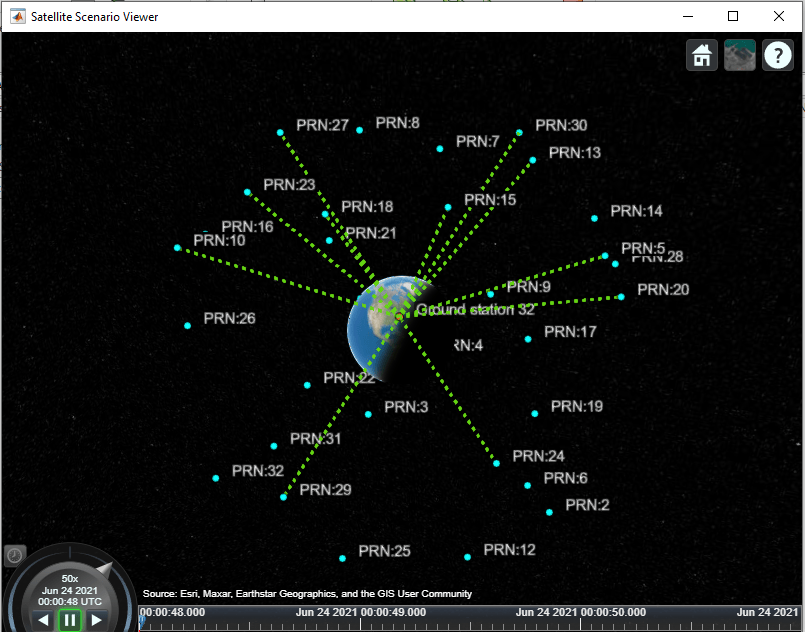```% Calculate Doppler shift over time for all the visible satellites fShift = dopplershift(sat(satindices),rx,Frequency=fe); % Get the states of satellites in the sky over time. Also, shuffle the % dimensions such that each row corresponds to one satellite data initsatpos = permute(states(sat,"CoordinateFrame","ecef"),[3 1 2]); % Compute the distance between satellites and receiver over time satdist = zeros(numsat,numSteps); for istep = 1:numSteps satdist(:,istep) = pseudoranges([rxlat,rxlon,rxel], ... initsatpos(satindices,:,istep),"RangeAccuracy",0); end delays = satdist/c; % Power at receiver from free space pathloss equation SqrtPr = sqrt(Pt*DtLin*DrLin)*(1./(4*pi*(fe+fShift).*delays)); timeinweek = mintow*6; PRNIDs = [navcfg(:).PRNID]; disp("Available satellites - " + num2str(PRNIDs(satindices)))```
```Available satellites - 5 10 13 15 18 20 23 24 27 29 30 ```

Generate C/A-codes for all the satellites in the GPS constellation. Use the codes for visible satellites while generating the waveform.

```cacodes = gnssCACode(PRNIDs,"GPS"); caCodeBlockDuration = 1e-3; % Constant value numcacodeblocks = stepTime/caCodeBlockDuration; % Each C/A-code block is of 1 millisecond duration % To rate match the C/A-code with P-code, repeat each element 10 times cacodesig1 = double(repelem(1-2*repmat(cacodes,numcacodeblocks,1),10,1)); % Rate match the C/A-code with the sampling rate [upfac,downfac] = rat(samplingRate/10.23e6); idx = 1:downfac:upfac*size(cacodesig1,1); cacodesig = cacodesig1(ceil(idx/upfac),:);```

Because P-code generation is a time-consuming process, and this example does not use the P-code for any receiver operations, use alternating values of `1` and `0` instead of the actual P-code. Divide the signal value by $\sqrt{2}$ because the I-branch on which P-code sits is attenuated by 3 dB as per IS-GPS-200 .

```numSamples = stepTime*samplingRate; pcode = (1-2*repmat([1;0],numSamples/2,1))/sqrt(2);```

Initialize the object needed to model the Doppler shift.

```pfo = comm.PhaseFrequencyOffset(FrequencyOffsetSource = "Input port", ... SampleRate = samplingRate);```

Initialize the object needed to model dynamic delays. The dynamic delay on the satellite signal is modeled in two steps. First, a static delay is modeled which does not change with time. Then, model the variable delay using `dsp.VariableFractionalDelay`. Initially while modeling the static delay, do not introduce the entire delay so that dynamic delay modeling handles the remaining delay.

```% Initialize static delay object dynamicDelayRange = 20000; staticdelay = round(delays(:,1)*samplingRate - dynamicDelayRange); if nnz(staticdelay<0)~=0 staticdelay = zeros(numsat,1); end staticDelayObj = dsp.Delay("Length",staticdelay); % Initialize Variable Fractional Delay object for modeling dynamic delay vfd = dsp.VariableFractionalDelay("InterpolationMethod","Farrow", ... "MaximumDelay",65535);```

Initialize the constellation diagram object.

```rxconstellation = comm.ConstellationDiagram(1,ShowReferenceConstellation=false, ... Title="Constellation diagram of signal at the output of tracking");```

Initialize the object that performs initial synchronization in the GPS receiver.

```initialsync = gnssSignalAcquirer; initialsync.SampleRate = samplingRate; initialsync.IntermediateFrequency = 0 % Baseband signal```
```initialsync = gnssSignalAcquirer with properties: GNSSSignalType: "GPS C/A" SampleRate: 10230000 IntermediateFrequency: 0 FrequencyRange: [-10000 10000] FrequencyResolution: 500 DetectionThresholdFactor: 1.9000 ```

Initialize the baseband file writer.

```if WriteWaveformToFile == 1 bbWriter = comm.BasebandFileWriter("gpsBBWaveform.bb",samplingRate,0); end```

Initialize the properties that are necessary for the end-to-end simulation chain.

```% Properties that store outputs of initial synchronization [doppleroffsets,codephoffsets] = deal(zeros(1,maxNumTrackingChannels)); % Properties required for storing outputs from tracking module [accuPh,accuFqy,accuFqyErr,accuPhErr,accuIntegWave,accuDelay,accuDelayErr] = ... deal(zeros(numSteps,maxNumTrackingChannels)); % Properties to store outputs of bit synchronization and frame % synchronization [maxTransitionLocation,sampleCounter] = deal(zeros(maxNumTrackingChannels,1)); syncidx = zeros(maxNumTrackingChannels,1); % Property to store output of data decoder deccfg = cell(maxNumTrackingChannels,1); % Initialize maximum number of steps for which the simulation chain runs maxSimSteps = 50/stepTime;```

### End-to-End Simulation Chain

This section contains three main steps:

1. Waveform generation

2. Propagation channel

As part of the propagation channel, model the Doppler offset, delay, and noise. As part of the receiver, the processes involved include initial synchronization, tracking, bit synchronization, frame synchronization, and data decoding.

```tic % Start of simulation for istep = 1:numSteps %% Generate waveform bitidx = floor((istep-1)/numCACodeBlocksPerBit)+1; allpcode = repmat(pcode,1,numsat); % Get navigation bit of each satellite at the corresponding step time navbitsig = 1-2*navdata(bitidx,satindices); iqsig = (allpcode + 1j*cacodesig(:,satindices)).*navbitsig; % Implicit expansion %% Propagation channel % Model the channel which models Doppler, delay, path loss, and noise on % the signal % Introduce Doppler to the signal as a frequency offset dopsig = pfo(iqsig,fShift(:,istep).'); % Introduce variable fractional delay staticDelayedSignal = staticDelayObj(dopsig); leftoutDelay = delays(:,istep)*samplingRate - staticdelay; % Value must always be positive delayedSig = vfd(staticDelayedSignal,leftoutDelay.'); % Scale the delayed signal as per received power calculated rmsPow = rms(delayedSig); rmsPow(rmsPow==0) = 1; % To avoid division by zero scaledsig = (SqrtPr(:,istep).').*delayedSig./rmsPow; % Add up all the signals at the receiver resultsig = sum(scaledsig,2); % Generate noise noisesig = (wgn(numSamples,1,10*log10(Nr)) + 1j*wgn(numSamples,1,10*log10(Nr)))./sqrt(2); % Add constant thermal noise to the composite signal rxwaveform = resultsig + noisesig; % Scale the received signal for having unit power waveform = rxwaveform/rms(rxwaveform); if WriteWaveformToFile == 1 bbWriter(waveform); end timeinweek = timeinweek + stepTime; %% Receiver % Because there are large delays (70 to 80 milliseconds) modeled on the % signal, start the receiver after some time only so as to process % valid signal instead of pure noise. if istep > rxWaitTime if performInitSync == 1 performInitSync = 0; [y,corrval] = initialsync(waveform,1:32); % Initial synchronization PRNIDsToSearch = y(y(:,4).IsDetected==1,1).PRNID.'; doppleroffsets = y(y(:,4).IsDetected==1,2).FrequencyOffset; codephoffsets = y(y(:,4).IsDetected==1,3).CodePhaseOffset; numdetectsat = length(PRNIDsToSearch); if numdetectsat > maxNumTrackingChannels % Set this value to limit the number of tracking channels numdetectsat = maxNumTrackingChannels; end % Perform the required initialization of tracking modules for each % channel and buffers to store the data. disp("The detected satellite PRN IDs: " + num2str(PRNIDsToSearch)) % Plot the correlation plot for the first satellite figure; mesh(-10e3:500:10e3, 0:size(corrval,1)-1, corrval(:,:,PRNIDsToSearch(1))); xlabel("Doppler Offset") ylabel("Code Phase Offset") zlabel("Correlation") msg = ["Correlation Plot for PRN ID: " num2str(PRNIDsToSearch(1))]; title(msg) framesyncbuffer = cell(1,numdetectsat); % Create a cell array, where each element corresponds to a carrier % tracking object. carrierCodeTrack = cell(numdetectsat,1); framesync = cell(numdetectsat,1); % Update properties for each tracking loop for isat = 1:numdetectsat carrierCodeTrack{isat} = HelperGPSCACodeCarrierTracker; carrierCodeTrack{isat}.SampleRate = samplingRate; carrierCodeTrack{isat}.CenterFrequency = 0; carrierCodeTrack{isat}.PLLNoiseBandwidth = PLLNoiseBW; carrierCodeTrack{isat}.FLLNoiseBandwidth = FLLNoiseBW; carrierCodeTrack{isat}.DLLNoiseBandwidth = DLLNoiseBW; carrierCodeTrack{isat}.PLLIntegrationTime = 1; % In milliseconds carrierCodeTrack{isat}.PRNID = PRNIDsToSearch(isat); carrierCodeTrack{isat}.InitialDopplerShift = doppleroffsets(isat); carrierCodeTrack{isat}.InitialCodePhaseOffset = codephoffsets(isat); % Initialize frame synchronization object framesync{isat} = HelperGPSLNAVFrameSynchronizer; end end % Because it would be sufficient to get receiver position after % running the simulation for 50 seconds of data, stop the loop % after executing for 50 seconds of data. In the default run of the % example, only 3 seconds of data is processed and this line is not % needed. This check is when running the example for large data % duration only (for at least 50 seconds of data). if istep > maxSimSteps break; end for isat = 1:numdetectsat % Perform tracking for each satellite [integwave,fqyerr,fqyoffset,pherr,phoffset,derr,dnco] = ... carrierCodeTrack{isat}(waveform); % Accumulate the values to see the results at the end accuFqyErr(rxcntr,isat) = fqyerr; accuFqy(rxcntr,isat) = fqyoffset; accuPhErr(rxcntr,isat) = pherr; accuPh(rxcntr,isat) = phoffset; accuIntegWave(rxcntr,isat) = sum(integwave); accuDelayErr(rxcntr,isat) = derr; accuDelay(rxcntr,isat) = dnco; end % Perform bit synchronization, frame synchronization, and data % decoding if numWaitingStepsForBitSync of receiver steps are % complete. if rxcntr > numWaitingStepsForBitSync % For each detected satellite, perform bit synchronization, % frame synchronization, and data decoding for isat = 1:numdetectsat if ~isBitSyncComplete(isat) maxTransitionLocation(isat) = ... gnssBitSynchronize( ... imag(accuIntegWave(1:numWaitingStepsForBitSync,isat)), ... numCACodeBlocksPerBit); isBitSyncComplete(isat) = 1; sampleCounter(isat) = rxcntr - maxTransitionLocation(isat) + 1; framesyncbuffer{isat} = accuIntegWave( ... maxTransitionLocation(isat):end,isat); else % Perform frame synchronization and data decoding sampleCounter(isat) = sampleCounter(isat) + 1; framesyncbuffer{isat}(sampleCounter(isat)) = accuIntegWave(rxcntr,isat); if mod(sampleCounter(isat),numStepsPerSubframe) == 0 samples = framesyncbuffer{isat}(sampleCounter(isat) - ... numStepsPerSubframe+1:sampleCounter(isat)); sym = mean(reshape(samples,numCACodeBlocksPerBit,[])); bits = imag(sym)<0; [syncidx(isat),rxsubframes,subframeIDs] = framesync{isat}(bits(:)); if ~isempty(rxsubframes) % Then perform data decoding deccfg{isat}.PRNID = PRNIDsToSearch(isat); deccfg{isat} = HelperGPSLNAVDataDecode(rxsubframes,deccfg{isat}); end end end end end if mod(rxcntr,1000) == 0 disp("Processed " + (rxcntr/1000) + " sec of data at the receiver.") rxconstellation(accuIntegWave(rxcntr-999:rxcntr,1)/ ... rms(accuIntegWave(rxcntr-999:rxcntr,1))) end % Update rxcntr rxcntr = rxcntr + 1; end end```
```The detected satellite PRN IDs: 13 24 18 27 5 20 23 15 29 10 30 ```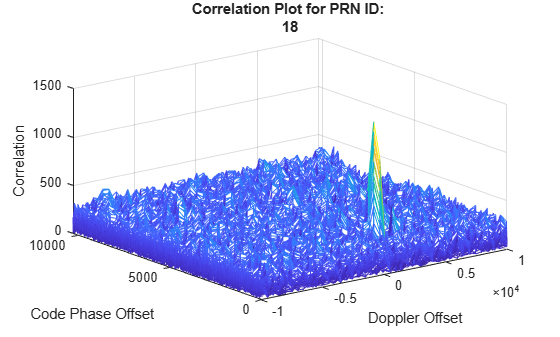```Processed 1 sec of data at the receiver. Processed 2 sec of data at the receiver. ```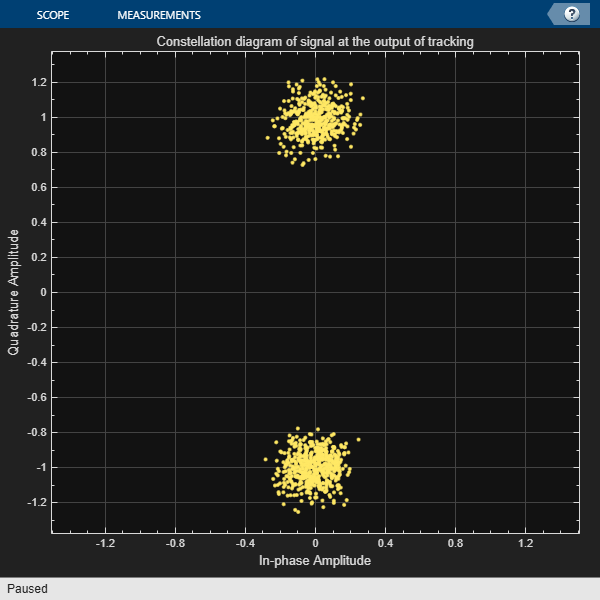```if WriteWaveformToFile == 1 release(bbWriter); end e2eTime = toc; disp("End-to-End chain ran for " + e2eTime + " seconds.")```
```End-to-End chain ran for 140.5149 seconds. ```

To estimate the receiver position, you need to know the distances from at least 4 satellites to the receiver and the location of each satellite in the sky at the time of transmission. Receiver position calculation from the output of data decoder involves these steps.

1. Estimate transmission time of visible satellites at given receiver time: Transmission time estimates are the natural measurements of a GPS receiver, not the pseudo-ranges. The transmission time of a signal is a culmination of timing computations performed at initial synchronization, tracking, bit synchronization, frame synchronization, and data decoding.

2. Compute pseudo-ranges of all satellites: Because the receiver clock is not accurate, you can expect large offsets in the estimated pseudo-ranges. For the same reason that these estimates are not true ranges, they are called pseudo-ranges.

3. Estimate visible satellites location in sky at given transmission time: From the decoded data, you get the ephemeris data. This ephemeris data is valid for 2-6 hours  and to calculate the accurate position of a satellite in the sky from this ephemeris data at a given time, use `gnssconstellation` (Navigation Toolbox).

4. Estimate receiver position: Estimate the receiver position using `receiverposition` (Navigation Toolbox).

Estimate the transmission time of visible satellites at a given receiver time.

```caChipRate = 1.023e6; % In Hz codeOffsetTime = codephoffsets(1:numdetectsat)/caChipRate; rxcntr(rxcntr<=1) = 2; % So that rxcntr-1 is at least 1 trackingOffsetTime = accuDelay(rxcntr-1,1:numdetectsat)/caChipRate; bitsyncTime = (maxTransitionLocation(1:numdetectsat) - 1)*caCodeBlockDuration; framesyncTime = (syncidx(1:numdetectsat)-1)*numCACodeBlocksPerBit*caCodeBlockDuration; % Calculate transmission time from these parameters tt1 = codeOffsetTime(:) - trackingOffsetTime(:) + bitsyncTime + framesyncTime; tt1 = tt1(syncidx~=0);```

Estimate the pseudo-ranges.

```trtemp = max(tt1); row = (tt1-trtemp)*c; % Include time of week decoded from the received navigation message tow = zeros(maxNumTrackingChannels,1); for isat = 1:maxNumTrackingChannels if isfield(deccfg{isat},"HOWTOW") tow(isat) = (deccfg{isat}.HOWTOW + 1)*6; % Each subframe has a duration of 6 seconds end end tow = tow(syncidx~=0); % Offset the estimated transmission time. This offset is found empirically. timeOffset = 0.74; tt = tt1 + tow + timeOffset;```

Estimate the satellite position at the computed transmission time.

```deccfg1 = deccfg(syncidx~=0); % Estimate the satellite position only if receiver has processed at least % minStepsForPosEst of data isFullDataDecoded = true(length(tt),1); if rxcntr > minStepsForPosEst [timeofweek,SatelliteID,Delta_n,M0,Eccentricity,sqrtA,Toe,Toc,Cis,Cic,Crs,Crc,Cus,Cuc, ... OMEGA0,i0,omega,OMEGA_DOT,IDOT,GPSWeek] = deal(zeros(4,1)); reqCEIFields = ["WeekNumber","ReferenceTimeOfEphemeris","ReferenceTimeOfClock", ... "SemiMajorAxisLength","MeanMotionDifference","MeanAnomaly","Eccentricity", ... "ArgumentOfPerigee","Inclination","InclinationRate","HarmonicCorrectionTerms", ... "RateOfRightAscension","LongitudeOfAscendingNode"]; for isat = 1:length(tt) timeofweek(isat) = tt(isat); isFullDataDecoded(isat) = (isfield(deccfg1{isat},"PRNID")) && ... (all(isfield(deccfg1{isat},reqCEIFields))); if isFullDataDecoded(isat) SatelliteID(isat) = deccfg1{isat}.PRNID; GPSWeek(isat) = deccfg1{isat}.WeekNumber; Toe(isat) = deccfg1{isat}.ReferenceTimeOfEphemeris; Toc(isat) = deccfg1{isat}.ReferenceTimeOfClock; sqrtA(isat) = sqrt(deccfg1{isat}.SemiMajorAxisLength); Delta_n(isat) = deccfg1{isat}.MeanMotionDifference*pi; M0(isat) = deccfg1{isat}.MeanAnomaly*pi; Eccentricity(isat) = deccfg1{isat}.Eccentricity; omega(isat) = deccfg1{isat}.ArgumentOfPerigee*pi; i0(isat) = deccfg1{isat}.Inclination*pi; IDOT(isat) = deccfg1{isat}.InclinationRate*pi; hterms = num2cell(deccfg1{isat}.HarmonicCorrectionTerms); [Cis(isat),Cic(isat),Crs(isat),Crc(isat),Cus(isat),Cuc(isat)] = deal(hterms{:}); OMEGA_DOT(isat) = deccfg1{isat}.RateOfRightAscension*pi; OMEGA0(isat) = deccfg1{isat}.LongitudeOfAscendingNode*pi; end end transmissionTime = HelperGPSConvertTime(GPSWeek(isFullDataDecoded),timeofweek(isFullDataDecoded)); refTime = HelperGPSConvertTime(GPSWeek(:),Toc(:)); rxtimetable = timetable(refTime,SatelliteID,Delta_n,M0,Eccentricity,sqrtA, ... Toe,Cis,Cic,Crs,Crc,Cus,Cuc,OMEGA0,i0,omega, ... OMEGA_DOT,IDOT,GPSWeek); [satpos, satvel] = gnssconstellation(transmissionTime(1),rxtimetable); end```

Estimate the receiver position, which requires two inputs — pseudo-ranges and the exact satellite position at the transmission time which is estimated at a given receiver time. You can compute these values only if the example runs for at least 48 seconds of data. Because simulating such data would take a long time, the pseudo-ranges and satellite position properties (obtained by running the example for 50 seconds) are stored in the MAT files. If you run the simulation for less than `minStepsForPosEst`, then these MAT files are loaded and the receiver position is computed. If you do not, the required properties are taken directly from the simulation chain.

```if rxcntr <= minStepsForPosEst % The parameters that are loaded here are valid for the default % configuration of this example load receiverPositionProperties; % When loading the parameters for default configuration, update the % isFullDataDecoded variable with all ones to compute the receiver % position. isFullDataDecoded = true(length(row),1); defaultRINEXFileName = "GODS00USA_R_20211750000_01D_GN.rnx"; defaultRxPos = [39.021, -76.827, 19]; exampleRxPos = [rxlat, rxlon, rxel]; if ~(strcmp(rinexFileName,defaultRINEXFileName) && isequal(defaultRxPos,exampleRxPos)) warning("satcom:EndToEndGPSLNAVReceiverExample:InsufficientData", ... "Estimated receiver position may be different from what you provided" + ... " as the simulation didn't run for entire data." + ... " To get accurate receiver position, run the example" + ... " for at least 50 seconds of navigation data."); end end % Compute the receiver position rxposest = receiverposition(row(isFullDataDecoded),satpos(isFullDataDecoded,:))```
```rxposest = 1×3 39.0211 -76.8270 21.2084 ```
```estRxPosNED = lla2ned(rxposest,[rxlat,rxlon,rxel],'ellipsoid'); distanceError = vecnorm(estRxPosNED) % In meters```
```distanceError = 7.5333 ```

### Further Exploration

This example shows how to perform GPS receiver processing for the simulated satellite constellation for only 3 seconds. Extend the example to 50 seconds and estimate the receiver position to see how the GPS receiver works.

Use your own RINEX file to configure the example and estimate the receiver position from the waveform generated in this example.

### Appendix

This example uses these data and helper files:

### References

 IS-GPS-200, Rev: M. NAVSTAR GPS Space Segment/Navigation User Interfaces. May 21, 2021; Code Ident: 66RP1.

 Elliott D. Kaplan and C. Hegarty, eds., Understanding GPS/GNSS: Principles and Applications, Third edition, GNSS Technology and Applications Series (Boston ; London: Artech House, 2017).